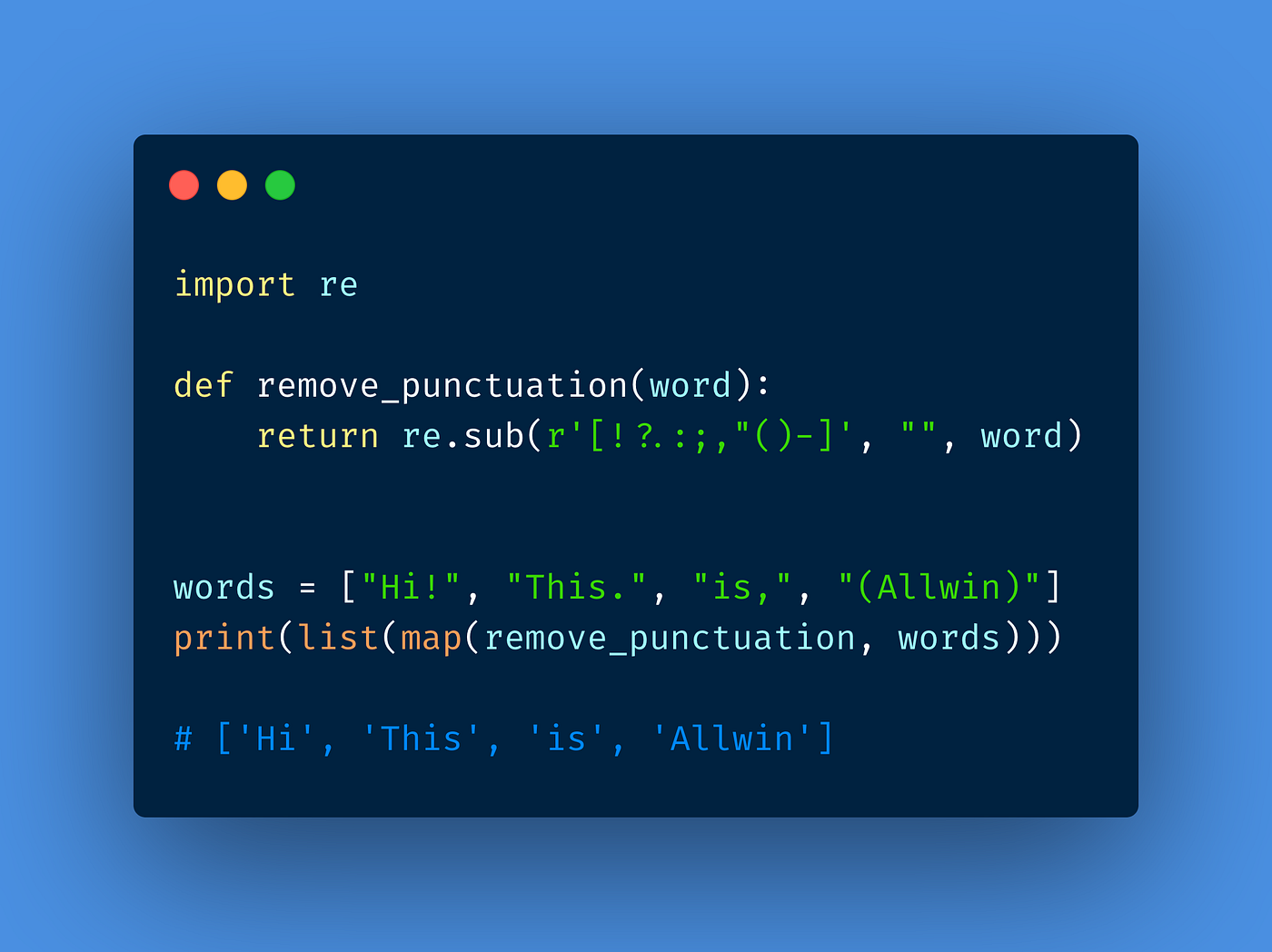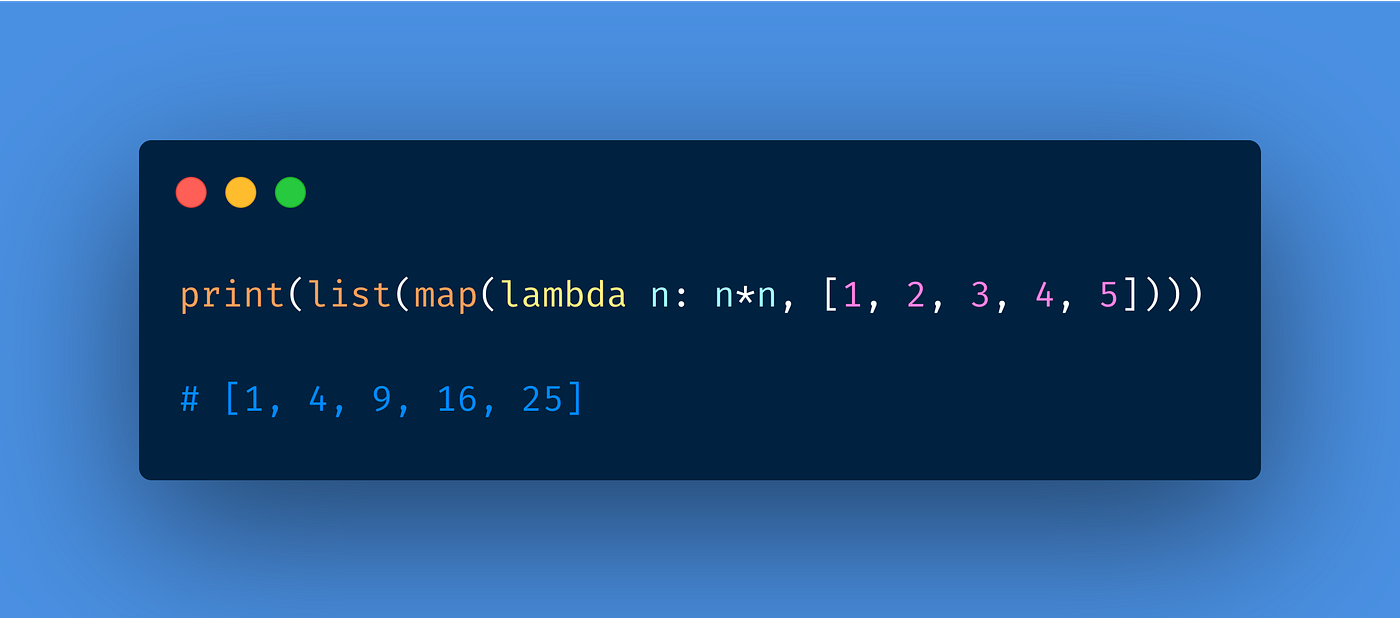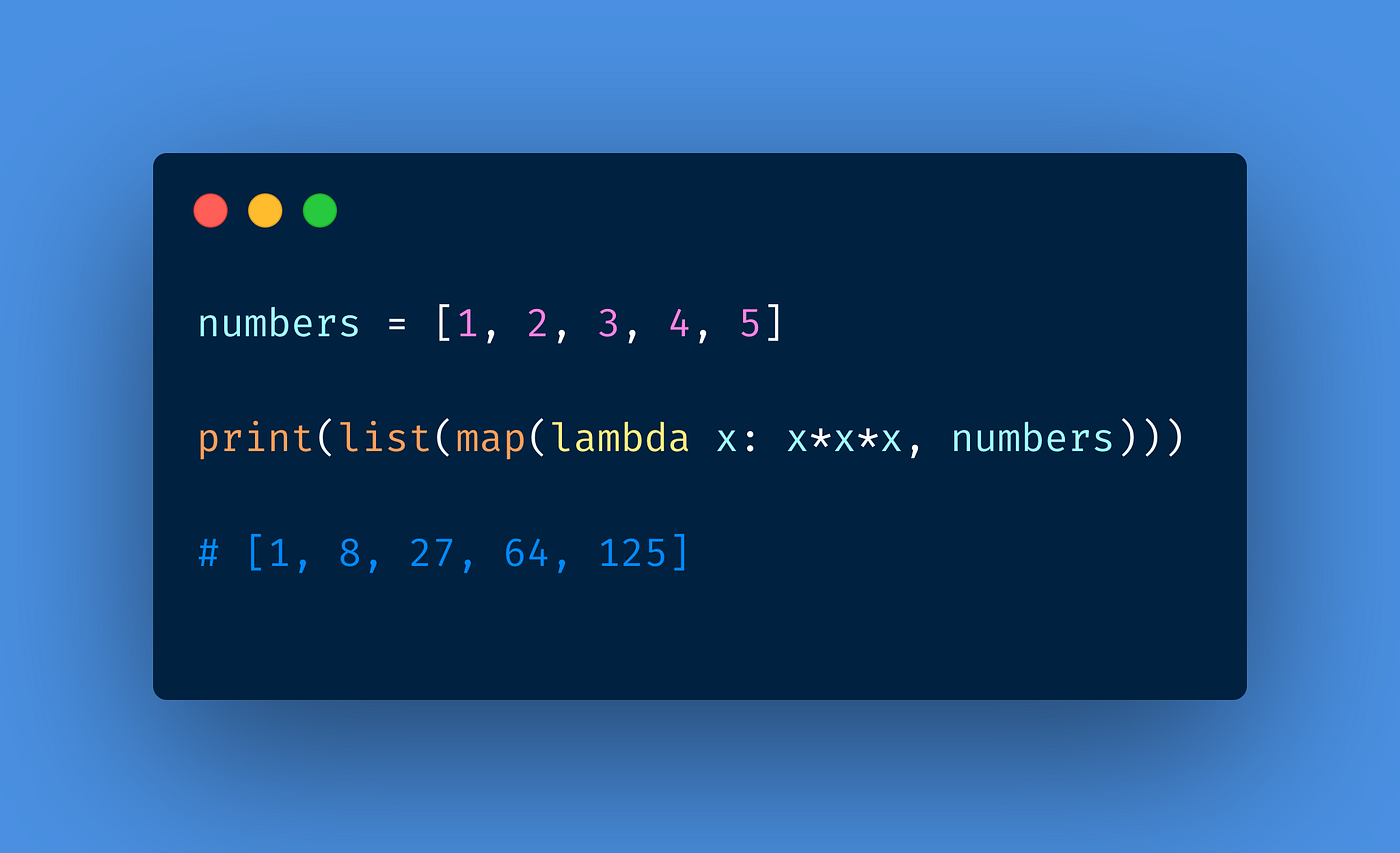# Cool things you can do with map in pythonPhoto by Marjan Blan | @marjanblan on Unsplash

## What is a map() function?

The map() function will take two arguments one a function and another an iterable (list, tuple, etc), and performs the function passed to each item of the iterable and returns a map object.

The map object can take different types of functions. They are

1. A normal function
2. A lambda function
3. A built-in function
4. A filter function

The main purpose of using the map function is to perform operations on the iterables without manually having to iterate over them.

Let us look at some of the cool things we can do with map functions with different types of functions.

# Map with normal functions

## Square the numbers in the list using a normal function

In this example, we are calculating the square of a number using a separate function and returning it. The map() function will take this function as its first argument.

## Remove punctuations with the map function

This example has a custom function that will take a string, remove all the punctuations in the string and return the string.Removing punctuations using the map() function

# Map with lambda functions

A lambda function is a small anonymous function. A lambda function can take any number of arguments, but can only have one expression.

The syntax for a lambda function is,

`lambda arguments : expression`

## Square the numbers in the list using a lambda function

This example is similar to the first example but with a lambda function. This is more of a one-liner code.square numbers using map and lambda functioncube numbers using map and lambda function

## Map function with two arguments

If we pass n sequences to map(), the function must take n number of arguments, and items from the sequences are consumed in parallel until the shortest sequence is exhausted.

# Map function with built-in functions

We can also map() function with some of the built-in functions such as abs, round, len, etc.

## Change the data type of the values

This code will take a list of numbers as strings and convert them to integers. This will come in handy when you are receiving input from the user for a list of integers.

## Find the length of the string

We can also pass built-in functions to the map function. In this example, I have a list of strings. I need to find the length of each string. I can do something like this.

## Using math functions with the map function

I can also import modules and use the methods provided by the module. In this example, I have used the ceil method from the math module to convert the list of integers to their next highest integer.

## Round the values using the map function

In this example, I have used the round method to round the list of floating numbers to their closest previous integer value.

## Generate a list of numbers

I can also generate a list of integers between any two values using the range method. In this example, I have generated a list of integers from 1 to 20 using the map() and range function.

## Get absolute value of the numbers

In this example, I have used the abs method to convert all the numbers to a positive integer.

# Map with filter

Sometimes you need to process an input iterable and return another iterable that results from filtering out unwanted values in the input iterable. In that case, Python’s filter() can be a good option for you. filter() is a built-in function that takes two positional arguments:

1. The function will be a predicate or Boolean-valued function, a function that returns True or False according to the input data.
2. Iterable will be any Python iterable.

## Square of positive integers using map and filter

In this example, I am filtering only the positive integers first using the filter method and then finding the square of only those positive integers.